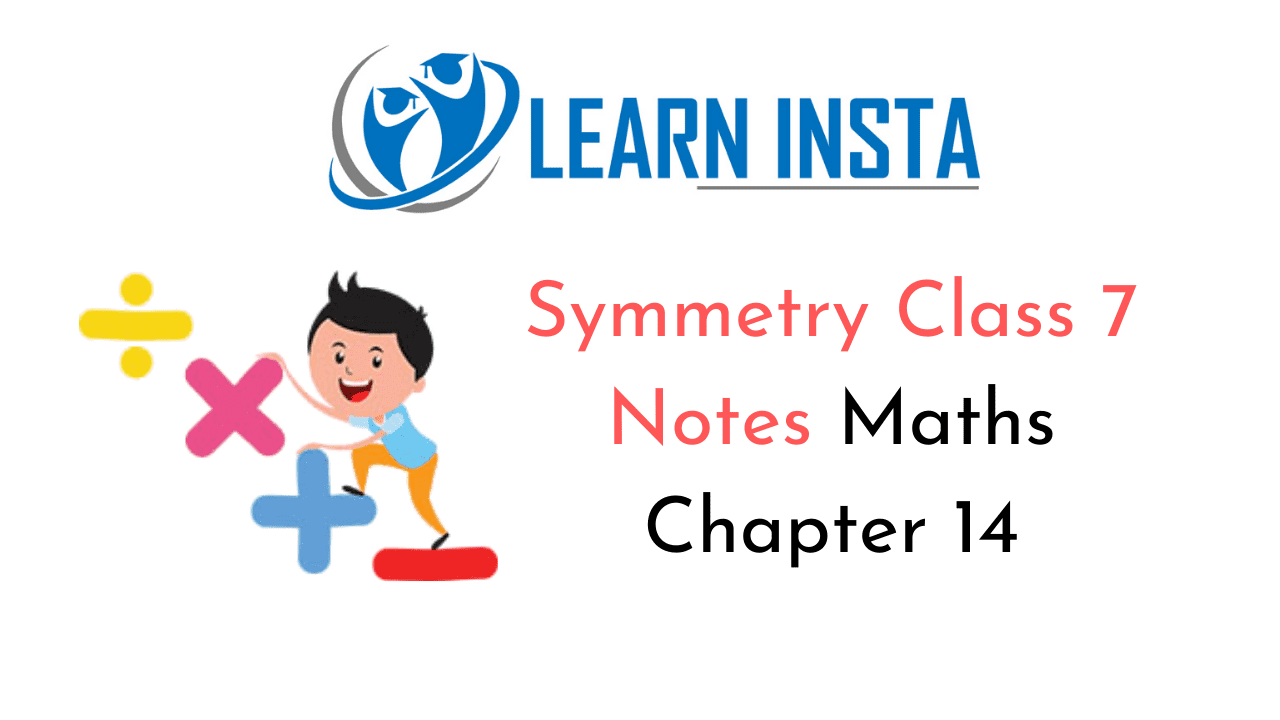On this page, you will find Symmetry Class 7 Notes Maths Chapter 14 Pdf free download. CBSE NCERT Class 7 Maths Notes Chapter 14 Symmetry will seemingly help them to revise the important concepts in less time.

## CBSE Class 7 Maths Chapter 14 Notes Symmetry

### Symmetry Class 7 Notes Conceptual Facts

Symmetry; If a paper is folded in half and the two halves of the paper exactly cover each other, then the shape of the paper is symmetric.
For example:Axis of symmetry: When a figure is folded in half then the line of fold is called axis of symmetry.
For example: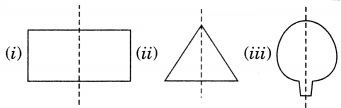Symmetry of regular polygons:Note: Each regular polygon has a many lines of symmetry as it has sides.

Mirror reflection symmetry: The symmetry in which one half of the shape is the image of the other.
For example: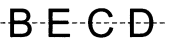Rotational symmetry: When an object rotate clockwise or anticlockwise about a fixed point and when it looks after some rotation by a partial turn then it is called rotational symmetry. This fixed point is known as centre of rotation.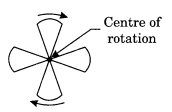Axis of rotation: The line of symmetry about of which an object rotates is called the axis of rotation.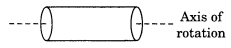Angle of rotation: The angle through which an object rotates is called angle of rotations.

• A half-turn means rotation by 180°.
• A quarter-turn means rotations by 90°.
• A complete-turn means rotation by 360°.

Order of rotational symmetry: If x° be the smallest angle through which a figure can rotate and still looks the same, then the order of rotational symmetry $$=\left(\frac{360}{x}\right)$$
For example:
(i) Order of square $$=\frac{360}{90}=4$$
(ii) Order of equilateral triangle $$=\frac{360}{90}=6$$